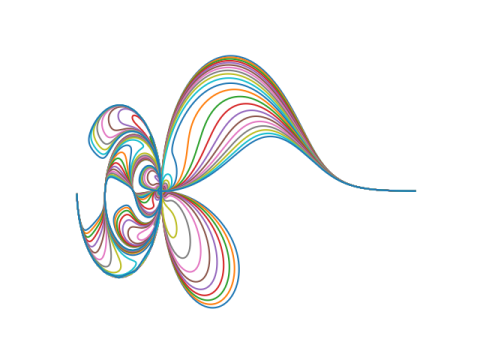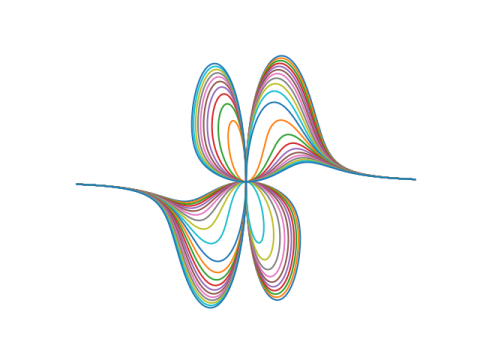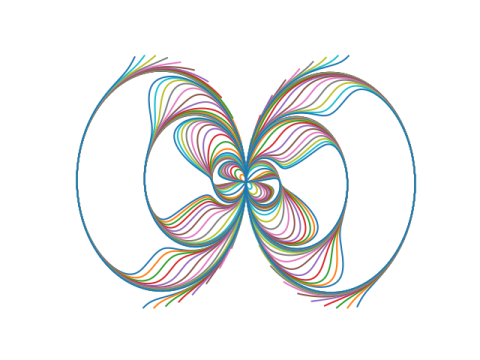# Some mathematical art

This evening I ran across a paper on an unusual coordinate system that creates interesting graphs based from simple functions. It’s called “circular coordinates,” but this doesn’t mean polar coordinates; it’s more complicated than that. 

Here’s a plot reproduced from , with some color added (the default colors matplotlib uses for multiple plots).The plot above was based on a the gamma function. Here are a few plots replacing the gamma function with another function.

Here’s x/sin(x):Here’s x5:And here’s tan(x):Here’s how the plots were created. For a given function f, plot the parametric curves given bySee  for what this has to do with circles and coordinates.

The plots based on a function g(x) are given by setting f(x) = g(x) + c where c = -10, -9, -8, …, 10.

## Related posts

 Elliot Tanis and Lee Kuivinen, Circular Coordinates and Computer Drawn Designs. Mathematics Magazine. Vol 52 No 3. May, 1979.

## 2 thoughts on “Some mathematical art”

1. Is there a typo in your post, or have I misunderstood the maths?

You say at teh end of the post

“The plots based on a function g(x) are given by setting f(x) = g(x) + c…”

Should that not be “f(x) = g(x) * c” – ie it is times c, not plus c

Or have I got the maths wrong?

PS I’m trying to play around with this in R

2. It is addition, g(x) + c, not multiplication.